GeeksforGeeks App
Open AppBrowser
Continue

## Related Articles

Bokeh is a library of Python which is used to create interactive data visualizations. In this article, we will discuss glyphs in Bokeh. But at first let’s see how to install Bokeh in Python.

## Installation

To install this type the below command in the terminal.

```conda install bokeh
Or
pip install bokeh  ```

## Plotting with glyphs

Usually, a plot consists of geometric shapes either in the form of a line, circle, etc. So, Glyphs are nothing but visual shapes that are drawn to represent the data such as circles, squares, lines, rectangles, etc.

### Creating a basic line chart:

The line chart displays the visualization of x and y-axis points movements in the form of a line. To draw a line glyph to the figure, we use the line() method of the figure object.

Syntax:

` my_plot.line(a, b, line_width)  `

Code:

## Python

 `# import the libraries``from` `bokeh.plotting ``import` `figure, show, output_file` `# prepare some data``a ``=` `[``1``, ``2``, ``3``, ``4``, ``5``, ``6``, ``7``, ``8``, ``9``, ``10``]``b ``=` `[``2``, ``4``, ``6``, ``8``, ``10``, ``12``, ``14``, ``16``, ``18``, ``20``]` `# create a plot using figure``my_plot ``=` `figure(title``=``"simple line chart"``, x_axis_label``=``"X-Axis"``,``                 ``y_axis_label``=``"Y-Axis"``)` `# adding line graph``my_plot.line(a, b, line_width``=``3``)` `# display output in file``output_file(``"line.html"``)` `# show the result``show(my_plot)`

Output: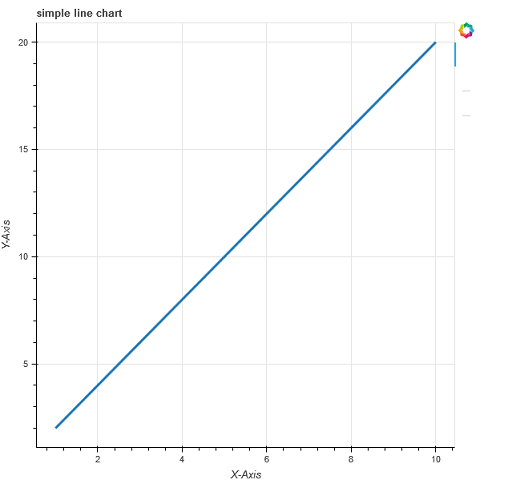### Combining multiple graphs

You can also add multiple graphs with the use of bokeh.plotting interface. To do so, you just need to call the line() function multiple times by passing different data as parameters as shown in the example.

Syntax:

`p.line(x1, y2, legend_label, line_color, line_width)`

Code:

## Python

 `from` `bokeh.plotting ``import` `figure, show``from` `bokeh.io ``import` `output_notebook`  `# prepare some data``x1 ``=` `[``1``, ``3``, ``4``, ``5``, ``6``]``x2 ``=` `[``5``, ``3``, ``8``, ``1``, ``8``]``y1 ``=` `[``6``, ``7``, ``8``, ``9``, ``4``]``y2 ``=` `[``3``, ``4``, ``5``, ``6``, ``7``]` `# create a new plot``p ``=` `figure(title``=``"Drawing multiple lines"``,``           ``x_axis_label``=``"X-Axis"``, y_axis_label``=``"Y-Axis"``)` `# add multiple renderers``p.line(x1, y1, legend_label``=``"line 1"``, line_color``=``"red"``, line_width``=``1``)``p.line(x2, y2, legend_label``=``"line 2"``, line_color``=``"blue"``, line_width``=``1``)``p.line(x1, y2, legend_label``=``"line 3"``, line_color``=``"black"``, line_width``=``1``)` `output_notebook()``show(p)`

Output: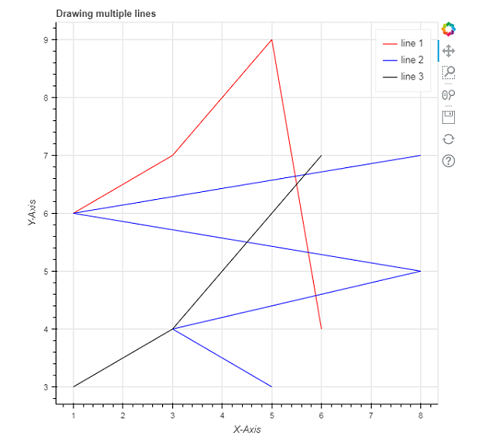## Rendering Circles

In order to add the circle glyph to your plot, we use the circle() method instead of the line() method used in the above example.

Circle(): We use this method to add a circle glyph to the plot. It takes x and y coordinates of the center as parameters. Apart from these, it takes parameters such as size, fill_color,fill_alpha,angle, line_color, line_alpha,radius,radius_dimensions,etc

Syntax:

`p.circle(x, y, size, fill_color)`

Criss_cross(): this method adds a circle glyph with a ‘+’ mark through the center of the circle and it takes x and y coordinates of the center.

Syntax:

p.circle_cross(x, y, size, fill_color, fill_alpha, line_width)

Circle_X(): This method adds a circle glyph with an ‘X’ mark through the center of the circle and it takes x and y coordinates of the center.

Syntax:

p.circle_x(x, y, size,fill_color, fill_alpha, line_width)

Code:

## Python

 `from` `bokeh.plotting ``import` `figure, show``from` `bokeh.io ``import` `output_file` `# prepare some data``x ``=` `[``1``, ``2``, ``4``, ``6``, ``7``]``y ``=` `[``7``, ``6``, ``3``, ``9``, ``10``]` `# create a new plot with figure function``p ``=` `figure(title``=``"Circle Glyph"``, plot_width``=``450``, plot_height``=``400``)` `# create circle glyph``p.circle(x``=``x, y``=``y, size``=``25``, fill_color``=``"red"``)``p.circle_cross(x``=``[``2``, ``4``, ``6``, ``8``], y``=``[``5``, ``8``, ``9``, ``11``], size``=``25``,``               ``fill_color``=``"blue"``, fill_alpha``=``0.3``, line_width``=``2``)``p.circle_x(x``=``[``4``, ``7``, ``2``, ``6``], y``=``[``7``, ``2``, ``4``, ``9``], size``=``25``,``           ``fill_color``=``"green"``, fill_alpha``=``0.6``, line_width``=``2``)` `# show the results``output_file(``'circle.html'``)``show(p)`

Output: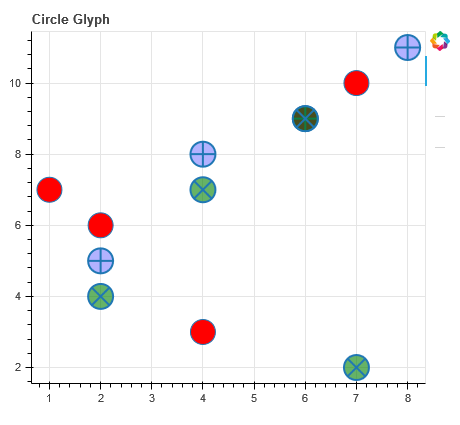## Rendering Bars

Similarly, we can render bars using vbar() function for vertical bars and hbar() function for horizontal bars.

### Creating vbar

To draw vbar, we specify center x-coordinate, bottom, and top endpoints as shown in the below example:

Syntax:

```p.vbar(x, bottom, top,
color, width, fill_color,legend_label)```

Code:

## Python

 `# Bokeh libraries``from` `bokeh.io ``import` `output_notebook``from` `bokeh.plotting ``import` `figure, show` `# data``day ``=` `[``1``, ``2``, ``3``, ``4``, ``5``, ``6``, ``7``, ``8``, ``9``, ``10``]``no_orders ``=` `[``450``, ``628``, ``488``, ``210``, ``287``,``             ``791``, ``508``, ``639``, ``397``, ``943``]` `# Output the visualization directly in the notebook``output_notebook()` `# Create a figure``p ``=` `figure(title``=``'Bar chart'``,``           ``plot_height``=``400``, plot_width``=``600``,``           ``x_axis_label``=``'Day'``, y_axis_label``=``'Orders Received'``,``           ``x_minor_ticks``=``2``, y_range``=``(``0``, ``1000``),``           ``toolbar_location``=``None``)` `# The daily orders will be represented as vertical``# bars (columns)``p.vbar(x``=``day, bottom``=``0``, top``=``no_orders,``       ``color``=``'blue'``, width``=``0.75``, fill_color``=``'red'``,``       ``legend_label``=``'Orders'``)` `# Let's check it out``show(p)`

Output: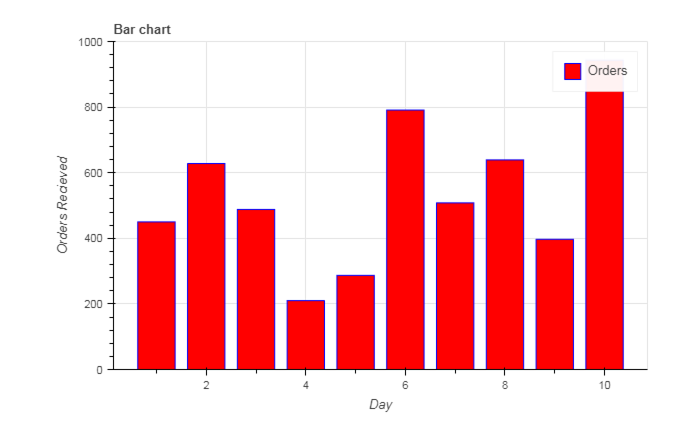### Creating hbar

To draw hbar , we specify center y-coordinate, left and right endpoints, and height as shown in the below example:

Syntax:

```p.hbar(y, height, left, right,
color, width, fill_color,
legend_label)```

## Python

 `# Bokeh libraries``from` `bokeh.plotting ``import` `figure, show, output_file` `# data``day ``=` `[``1``, ``2``, ``3``, ``4``, ``5``, ``6``, ``7``, ``8``, ``9``, ``10``]``no_orders ``=` `[``450``, ``628``, ``488``, ``210``, ``287``, ``791``,``             ``508``, ``639``, ``397``, ``943``]` `# Create a figure``p ``=` `figure(title``=``'Bar chart'``,``           ``plot_height``=``400``, plot_width``=``600``,``           ``x_axis_label``=``'Orders Received'``, y_axis_label``=``'Day'``,``           ``x_range``=``(``0``, ``1000``), toolbar_location``=``None``)` `# The daily orders will be represented as``# horizontal bars``p.hbar(y``=``day, height``=``0.5``, left``=``0``, right``=``no_orders,``       ``color``=``'blue'``, width``=``0.75``, fill_color``=``'red'``,``       ``legend_label``=``'Orders'``)` `# Let's check it out``show(p)``output_file(``"ex.html"``)`

Output: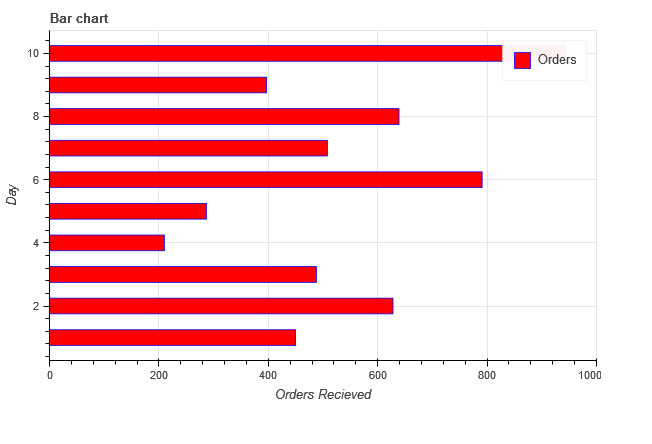## Patch Glyph

The patch glyph shades a region of space in a particular color. We use the patch() method to develop a single patch and patches() method to develop multiple patches.

### Single patch

Syntax:

`p.patch(x, y, fill_color, line_color, alpha, line_width)`

Code:

## Python

 `# Bokeh libraries``from` `bokeh.plotting ``import` `figure, show, output_file` `# data``x ``=` `[``1``, ``2``, ``3``, ``4``, ``5``]``y ``=` `[``6``, ``7``, ``8``, ``5``, ``2``]` `# Create a figure``p ``=` `figure(title``=``'Patch Glyph'``,``           ``plot_height``=``400``, plot_width``=``600``,``           ``x_axis_label``=``'x'``, y_axis_label``=``'y'``,``           ``toolbar_location``=``None``)` `# add patch glyph``p.patch(x, y, fill_color``=``"blue"``, line_color``=``'black'``,``        ``alpha``=``0.5``, line_width``=``2``)` `# show the results``show(p)``output_file(``"ex.html"``)`

Output: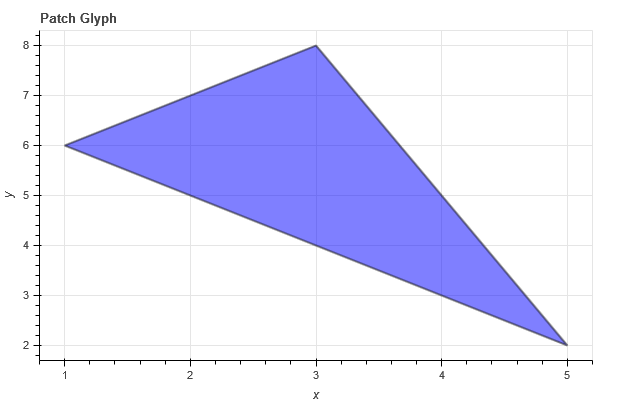### Multiple Patches

Multiple patches can be created in a similar way by using the function patches() instead of patch(). We are passing the data in the form of a list of arrays for creating three patches with different colors in the example shown below.

Code:

## Python

 `# Bokeh libraries``from` `bokeh.plotting ``import` `figure, show, output_file` `# data``x ``=` `[[``1``, ``2``, ``3``], [``4``, ``6``, ``8``], [``2``, ``4``, ``5``, ``4``]]``y ``=` `[[``2``, ``5``, ``6``], [``3``, ``6``, ``7``], [``2``, ``4``, ``7``, ``8``]]` `# Create a figure``p ``=` `figure(title``=``'Patch Glyph'``,``           ``plot_height``=``400``, plot_width``=``600``,``           ``x_axis_label``=``'x'``, y_axis_label``=``'y'``,``           ``toolbar_location``=``None``)` `# add patches glyph``p.patches(x, y, fill_color``=``[``"blue"``, ``"green"``, ``"yellow"``], line_width``=``2``)` `# show the results``show(p)``output_file(``"ex.html"``)`

Output: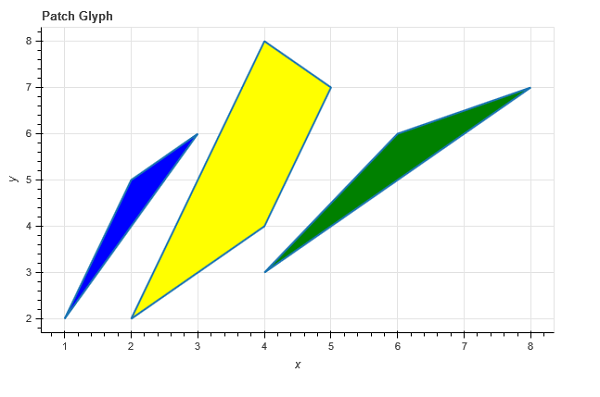My Personal Notes arrow_drop_up